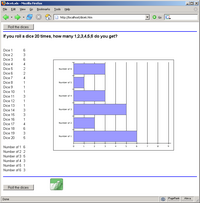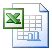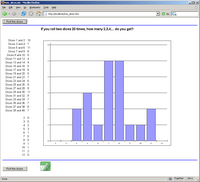This is an example of what you can do with SpreadsheetConverter, a clever program that lets you publish your Excel spreadsheets on the web. Send us one of your own spreadsheets and we’ll convert it to a web page for you, for free!

Visualizing probability online on a web page using SpreadsheetConverter with it’s chart support is easy. In this example we use bar charts and column charts to visualize the outcome of rolling dice.

The following spreadsheet shows the outcome of rolling ONE DIE 20 times using a histogram. Click on the image to open the calculator. Click on the Excel icon to download the Microsoft Excel spreadsheet used by SpreadsheetConverter to generate the webpage.Click to roll a single dieExcel spreadsheet for rolling a single die

The following spreadsheet shows the outcome of rolling A PAIR OF DICE 20 times using a histogram. Click on the image to open the calculator. Click on the Excel icon to download the Microsoft Excel spreadsheet used by SpreadsheetConverter to generate the webpage.Click to roll a pair of diceExcel spreadsheet for rolling a pair of dice

Probability and Dice

Rolling Dice

Statistics and Dice

Risk

Risk Board Game Dice Odds

Risk dice odds

An Optimal Dice Rolling Policy for Risk

Settlers of Catan

Dice Odds for Settlers of Catan, pair of dice

Monopoly

Monopoly Probabilities